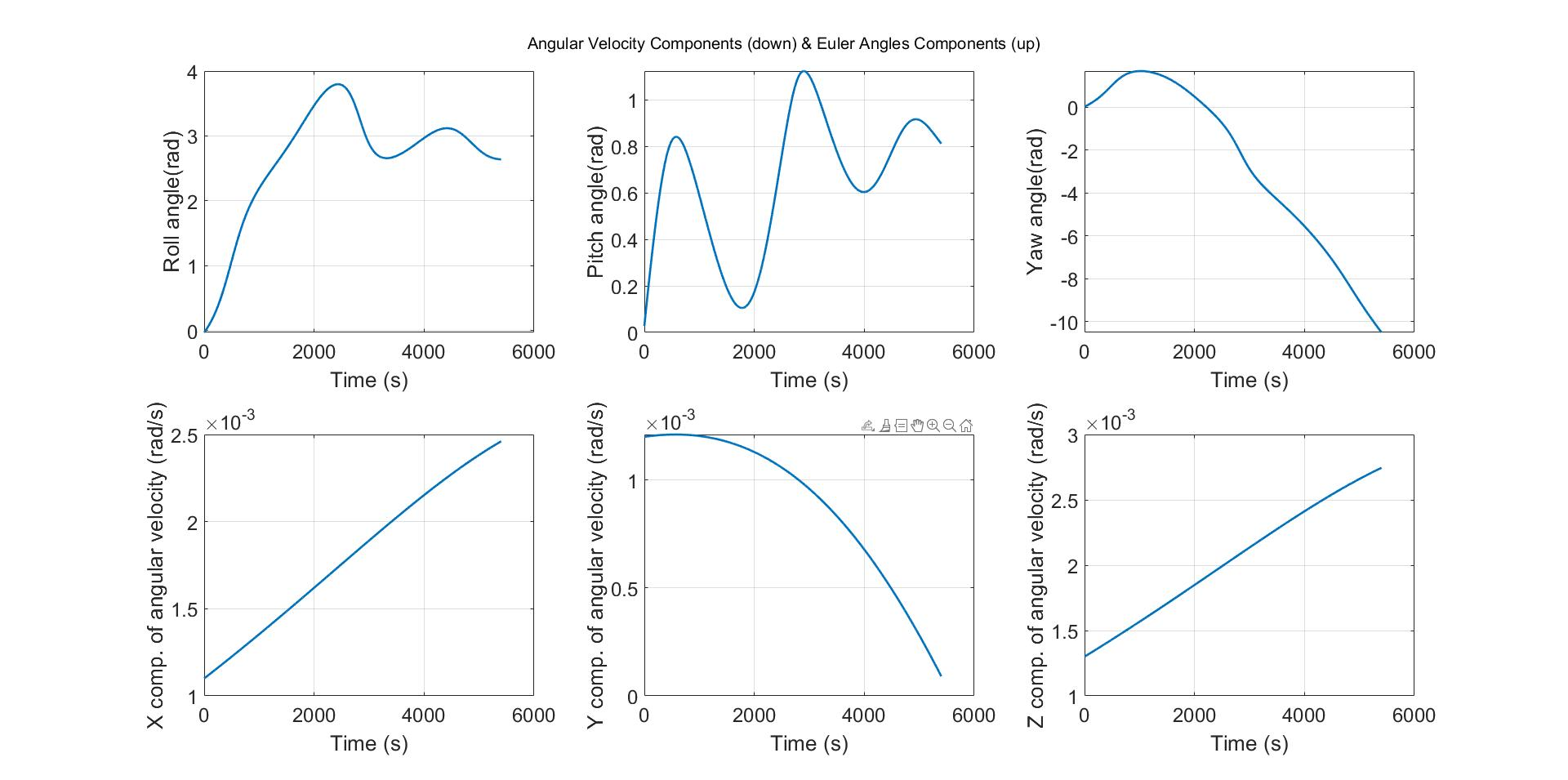## Simulation of the LEO Satellite’s Attitude Dynamics

Version 1.0.0 (1.88 KB) by
The subject of the program is to characterize the attitude dynamics of the Low Earth Orbit satellite in the simulation environment.

Updated 6 Jul 2022

• The subject of the program is to characterize the attitude dynamics of the Low Earth Orbit satellite in the simulation environment. Since euler angles are correlated with angular velocity components, firstly, the angular velocity values will be found with the given initial values and then the values found will be transferred to the vectors. This matrix data and euler angles will be found using the initial data and finally presented as a plot graph.
• -𝝓 is the roll angle, -𝜽 is the pitch angle, -𝝍 is the yaw angle, -𝒘𝒙, 𝒘𝒚, and 𝒘𝒛 are the angular velocities of the satellite, -𝑱𝒙, 𝑱𝒚, and 𝑱𝒛 are the moments of inertia of the satellite, -𝒘𝒐𝒓𝒃𝒊𝒕 is the angular orbit velocity of the satellite, -𝑵𝑻 is the disturbance torque acting on the satellite, -𝑵 is the iteration number, -∆𝒕 is the sample time, -A is the transformation matrices.### Cite As

Anil Taha Balkir (2023). Simulation of the LEO Satellite’s Attitude Dynamics (https://www.mathworks.com/matlabcentral/fileexchange/114640-simulation-of-the-leo-satellite-s-attitude-dynamics), MATLAB Central File Exchange. Retrieved .

##### MATLAB Release Compatibility
Created with R2020a
Compatible with any release
##### Platform Compatibility
Windows macOS Linux